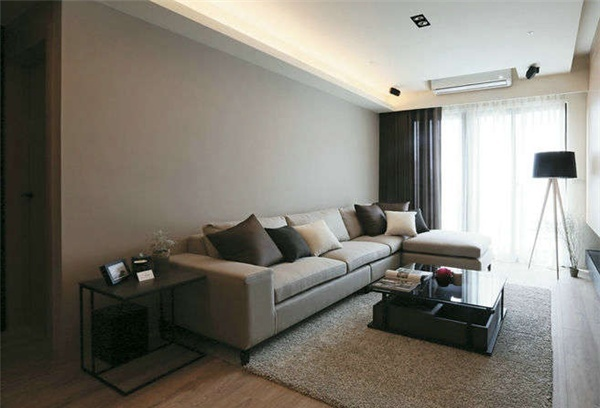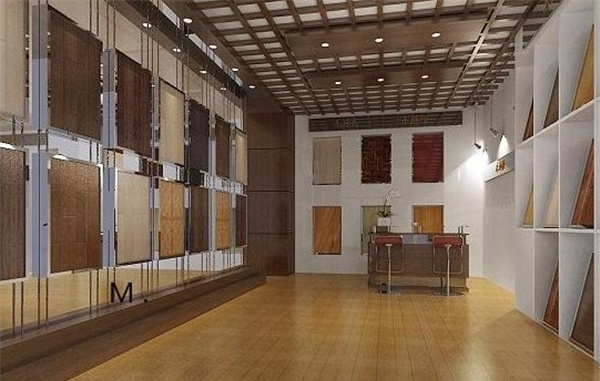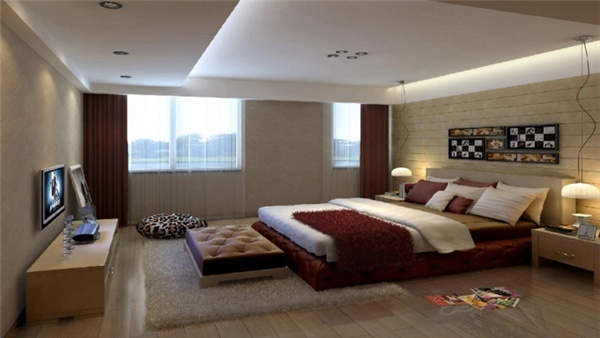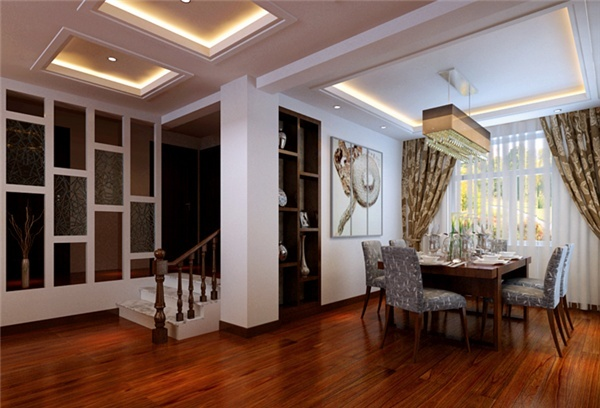|

# 冬季来临 更要注重地板保养措施

这一晃，又将步入寒冷的冬季，冬季是一个干燥寒冷的季节，特别容易的出现缺水的现象。人在冬季就需要特别的保养，当然室内家具中需要保养的地方也就会很多，就比如说家庭的地板就是需要一番精心养护的对象，尤其是在冬季这个寒冷的季节，就要更加注重保养的方式，这样才能够使地板的效果展现得最好。地板三大致命弱点

接缝起鼓

产生原因

地板表面进水：在清洁地板时或者在平时不小心造成地面积水，这时水就会顺着接接缝中进入，地板就会大量吸收水分，结果产生了局部膨胀或者起小泡的现象。

地板下面进水：这种现象主要放生在比较潮湿的地方，比如厨房、卫生间等，地板下层长期被湿气侵蚀，就会产生起鼓现象，而这种起鼓的形状就比较统一均匀。

修补方法：只可拆掉原来的旧地板，更换新的地板。

地板起拱

产生原因

伸缩缝预留不足：在铺设地板之时没有考虑到预留地板伸缩缝，地板有一个膨胀的过程，正因为伸缩缝不足所以导致了起拱的现象。

房间通铺：房间与房间之间安装地板时没有考虑到加装扣条，湿气与潮气在房间之间延伸，地板与地板之间互相牵扯，使地板起拱。

地面基层有异物：在对地面基层处理时，有异物遗留在地表没有及时处理干净，也会导致地板起拱的现象。

地板基层起拱：木地板本身因为受潮会出现变形，一旦变形就会影响地板的稳定性而发生起拱。

修补方法：找出起拱的问题所在，具有针对性的处理，伸缩缝预留要够，房间与房间之接合处安装扣条，有需要的要将地板起开重新进行补装。

地板裂缝

产生原因

地面不平：没有确保地面的平整性而进行地板安装将会导致胶水脱落产生缝隙。

施胶少：由于胶水施加得太多，在冬季比较干燥的气候里地板收缩加快，胶水不足会导致强度不够而产生裂缝。

重物施压：有重物的地方地板无法自由收缩，就会产生裂缝。

修补方法：将有裂缝的地板进行拆除，将地面整理平整后重新铺装；根据地板裂缝的大小进行灌胶或者补蜡；将重物挪开，使用灌胶的方法重新固定。要懂得防范于未然

水：作为地板的最大的一个克星，在日常生活中一定要谨慎，地板长期遭受到水的侵蚀会导致变形以及腐烂，所以一定要保持地板的干燥。

阳光：长期将地板暴露在阳光下，会导致地板变形褪色的现象，在必要时选择窗帘等遮阳的物品进行遮挡。

高温：地板受到高温的影响会出现起鼓变形的现象，所以在生活中尽量避免直接将取暖器等设备放置的地板上。

重物：过重的物品会对地板产生压制，地板无法自由伸缩，在放置重物家具时可以加上软垫进行保护地板，也避免地板被刮花，延长使用寿命。

虫蚁：地板最为容易招惹虫蚁，虫蚁对地板具有很大的破坏性，可以定期使用杀虫剂进行消除，或者是使用地板精油进行护理都可以。

化学剂：强性化学剂或者腐蚀剂等都不能够对地板使用，这样会破坏地板表层，影响地板使用寿命，一旦不慎洒在地板上要及时擦拭清除。地板清洁流程有讲究

工具准备

清洁工具：抹布、吸尘器、清洗液、白醋、软布。

保养工具：地板蜡。

防污工具：脱脂剂、25℃的温水。

基本清洁

1、利用吸尘器将地面上的脏物清除；

2、将清洗液与软布相结合，进行地面清洁；

3、有顽固污渍的地方可以使用白醋进行局部擦拭；

4、最后用干净的抹布保持地面的干燥即可。

保养处理

1、将地板蜡盛入容器并搅拌均匀；

2、为了防止地板蜡弄脏墙脚以及踢脚线，可用胶带纸进行遮盖；

3、用抹布沾取适量地板蜡顺着地板纹理方向进行仔细涂抹；

4、涂抹要保持均匀，切记不要太薄也不要太厚；

5、上好的蜡最好是静置1个小时，完全干燥即可。

防污方法

1、将脱脂剂与25℃的温水相融合；

2、使用软布沾取适量的溶剂进行清洁；

3、最后用干净的抹布擦拭地板表面的水分，保持地表的干燥性能。冬季保养两大要点

控制温、湿度

要点阐述

最适合地板的室内温度应该在18℃～24℃，室内控制在40％～80％之间，但是冬季是属于酷冷干燥的季节，对于温湿度的要求颇高。一旦室内温湿度被打破，那么地板就会很容易发生变形和其它破坏问题。

解决方案

在冬季室内要着重注意地暖的温度，室内温差不要过大，在缺少湿度的同时建议使用加湿器或者定期开窗通风的方法，简单直接有效，这样就能够充分的保持室内温湿度均衡。

针对性养护

要点阐述

在冬季最好是使用针对性比较强的养护方法，这样就能够减少地板的损伤，也能够减轻劳力物力以及财力。

解决方案

地板在冬季面临着最大的挑战就是失水过多而收缩，那么就要给地面进行一个“面膜”处理，那就是打蜡，地板每年最好进行两次打蜡，而其中的一次最好是选择在冬季，冬季打蜡是解决地板收缩这一问题的最好方法。

巧用小物品，显示大神力

精盐

精盐是我们常用到的调味品，当地板上有难处理的印迹时可以撒上精盐，静置十分钟后用抹布轻轻擦拭即可。

色拉油

油本来就有一个提亮的作用，在清洁地板时在水里加上些许色拉油不仅清洁地板更加的容易，还能够在地板附加上一层保护膜，地板也会显得亮堂堂。DIY清洁剂

市面上清洁剂较多，所含成分各有所异，在不懂得如何选择清洁剂的情况下可以尝试自己动手做，将软肥皂、漂白土、苏打和水以1：1：1：5的比例混合，放入大锅中煮沸，其中的液体就是非常好的清洁剂，清洁起来非常的得心应手。

自制地板蜡

家里有剩余的蜡烛头可以保留，收集到一定的数量后就可以自做地板蜡，将蜡烛头切碎，去掉烛芯，和松节油以1：1的比例混合，隔水煮沸，融化的蜡烛液体充分搅拌后倒入容器，冷却后就是一块不错的地板蜡。

结语：想要保养好自家的地板确实是要花一定的心思的，不过这些保养要点其实在我们平时生活中都可以做到的，既然都要做为什么不好好做呢？所以，对于地板的保养也并不是那么难。

`声明：本文由入驻焦点开放平台的作者撰写，除焦点官方账号外，观点仅代表作者本人，不代表焦点立场错误信息举报电话： 400-099-0099，邮箱：jubao@vip.sohu.com，或点此进行意见反馈，或点此进行举报投诉。`A B C D E F G H J K L M N P Q R S T W X Y Z
A - B - C - D - E
• A
• 鞍山
• 安庆
• 安阳
• 安顺
• 安康
• 澳门
• B
• 北京
• 保定
• 包头
• 巴彦淖尔
• 本溪
• 蚌埠
• 亳州
• 滨州
• 北海
• 百色
• 巴中
• 毕节
• 保山
• 宝鸡
• 白银
• 巴州
• C
• 承德
• 沧州
• 长治
• 赤峰
• 朝阳
• 长春
• 常州
• 滁州
• 池州
• 长沙
• 常德
• 郴州
• 潮州
• 崇左
• 重庆
• 成都
• 楚雄
• 昌都
• 慈溪
• 常熟
• D
• 大同
• 大连
• 丹东
• 大庆
• 东营
• 德州
• 东莞
• 德阳
• 达州
• 大理
• 德宏
• 定西
• 儋州
• 东平
• E
• 鄂尔多斯
• 鄂州
• 恩施
F - G - H - I - J
• F
• 抚顺
• 阜新
• 阜阳
• 福州
• 抚州
• 佛山
• 防城港
• G
• 赣州
• 广州
• 桂林
• 贵港
• 广元
• 广安
• 贵阳
• 固原
• H
• 邯郸
• 衡水
• 呼和浩特
• 呼伦贝尔
• 葫芦岛
• 哈尔滨
• 黑河
• 淮安
• 杭州
• 湖州
• 合肥
• 淮南
• 淮北
• 黄山
• 菏泽
• 鹤壁
• 黄石
• 黄冈
• 衡阳
• 怀化
• 惠州
• 河源
• 贺州
• 河池
• 海口
• 红河
• 汉中
• 海东
• 怀来
• I
• J
• 晋中
• 锦州
• 吉林
• 鸡西
• 佳木斯
• 嘉兴
• 金华
• 景德镇
• 九江
• 吉安
• 济南
• 济宁
• 焦作
• 荆门
• 荆州
• 江门
• 揭阳
• 金昌
• 酒泉
• 嘉峪关
K - L - M - N - P
• K
• 开封
• 昆明
• 昆山
• L
• 廊坊
• 临汾
• 辽阳
• 连云港
• 丽水
• 六安
• 龙岩
• 莱芜
• 临沂
• 聊城
• 洛阳
• 漯河
• 娄底
• 柳州
• 来宾
• 泸州
• 乐山
• 六盘水
• 丽江
• 临沧
• 拉萨
• 林芝
• 兰州
• 陇南
• M
• 牡丹江
• 马鞍山
• 茂名
• 梅州
• 绵阳
• 眉山
• N
• 南京
• 南通
• 宁波
• 南平
• 宁德
• 南昌
• 南阳
• 南宁
• 内江
• 南充
• P
• 盘锦
• 莆田
• 平顶山
• 濮阳
• 攀枝花
• 普洱
• 平凉
Q - R - S - T - W
• Q
• 秦皇岛
• 齐齐哈尔
• 衢州
• 泉州
• 青岛
• 清远
• 钦州
• 黔南
• 曲靖
• 庆阳
• R
• 日照
• 日喀则
• S
• 石家庄
• 沈阳
• 双鸭山
• 绥化
• 上海
• 苏州
• 宿迁
• 绍兴
• 宿州
• 三明
• 上饶
• 三门峡
• 商丘
• 十堰
• 随州
• 邵阳
• 韶关
• 深圳
• 汕头
• 汕尾
• 三亚
• 三沙
• 遂宁
• 山南
• 商洛
• 石嘴山
• T
• 天津
• 唐山
• 太原
• 通辽
• 铁岭
• 泰州
• 台州
• 铜陵
• 泰安
• 铜仁
• 铜川
• 天水
• 天门
• W
• 乌海
• 乌兰察布
• 无锡
• 温州
• 芜湖
• 潍坊
• 威海
• 武汉
• 梧州
• 渭南
• 武威
• 吴忠
• 乌鲁木齐
X - Y - Z
• X
• 邢台
• 徐州
• 宣城
• 厦门
• 新乡
• 许昌
• 信阳
• 襄阳
• 孝感
• 咸宁
• 湘潭
• 湘西
• 西双版纳
• 西安
• 咸阳
• 西宁
• 仙桃
• 西昌
• Y
• 运城
• 营口
• 盐城
• 扬州
• 鹰潭
• 宜春
• 烟台
• 宜昌
• 岳阳
• 益阳
• 永州
• 阳江
• 云浮
• 玉林
• 宜宾
• 雅安
• 玉溪
• 延安
• 榆林
• 银川
• Z
• 张家口
• 镇江
• 舟山
• 漳州
• 淄博
• 枣庄
• 郑州
• 周口
• 驻马店
• 株洲
• 张家界
• 珠海
• 湛江
• 肇庆
• 中山
• 自贡
• 资阳
• 遵义
• 昭通
• 张掖
• 中卫

1室1厅1厨1卫1阳台

1
2
3
4
5

0
1
2

1

1

0
1
2
3报名成功，资料已提交审核A B C D E F G H J K L M N P Q R S T W X Y Z
A - B - C - D - E
• A
• 鞍山
• 安庆
• 安阳
• 安顺
• 安康
• 澳门
• B
• 北京
• 保定
• 包头
• 巴彦淖尔
• 本溪
• 蚌埠
• 亳州
• 滨州
• 北海
• 百色
• 巴中
• 毕节
• 保山
• 宝鸡
• 白银
• 巴州
• C
• 承德
• 沧州
• 长治
• 赤峰
• 朝阳
• 长春
• 常州
• 滁州
• 池州
• 长沙
• 常德
• 郴州
• 潮州
• 崇左
• 重庆
• 成都
• 楚雄
• 昌都
• 慈溪
• 常熟
• D
• 大同
• 大连
• 丹东
• 大庆
• 东营
• 德州
• 东莞
• 德阳
• 达州
• 大理
• 德宏
• 定西
• 儋州
• 东平
• E
• 鄂尔多斯
• 鄂州
• 恩施
F - G - H - I - J
• F
• 抚顺
• 阜新
• 阜阳
• 福州
• 抚州
• 佛山
• 防城港
• G
• 赣州
• 广州
• 桂林
• 贵港
• 广元
• 广安
• 贵阳
• 固原
• H
• 邯郸
• 衡水
• 呼和浩特
• 呼伦贝尔
• 葫芦岛
• 哈尔滨
• 黑河
• 淮安
• 杭州
• 湖州
• 合肥
• 淮南
• 淮北
• 黄山
• 菏泽
• 鹤壁
• 黄石
• 黄冈
• 衡阳
• 怀化
• 惠州
• 河源
• 贺州
• 河池
• 海口
• 红河
• 汉中
• 海东
• 怀来
• I
• J
• 晋中
• 锦州
• 吉林
• 鸡西
• 佳木斯
• 嘉兴
• 金华
• 景德镇
• 九江
• 吉安
• 济南
• 济宁
• 焦作
• 荆门
• 荆州
• 江门
• 揭阳
• 金昌
• 酒泉
• 嘉峪关
K - L - M - N - P
• K
• 开封
• 昆明
• 昆山
• L
• 廊坊
• 临汾
• 辽阳
• 连云港
• 丽水
• 六安
• 龙岩
• 莱芜
• 临沂
• 聊城
• 洛阳
• 漯河
• 娄底
• 柳州
• 来宾
• 泸州
• 乐山
• 六盘水
• 丽江
• 临沧
• 拉萨
• 林芝
• 兰州
• 陇南
• M
• 牡丹江
• 马鞍山
• 茂名
• 梅州
• 绵阳
• 眉山
• N
• 南京
• 南通
• 宁波
• 南平
• 宁德
• 南昌
• 南阳
• 南宁
• 内江
• 南充
• P
• 盘锦
• 莆田
• 平顶山
• 濮阳
• 攀枝花
• 普洱
• 平凉
Q - R - S - T - W
• Q
• 秦皇岛
• 齐齐哈尔
• 衢州
• 泉州
• 青岛
• 清远
• 钦州
• 黔南
• 曲靖
• 庆阳
• R
• 日照
• 日喀则
• S
• 石家庄
• 沈阳
• 双鸭山
• 绥化
• 上海
• 苏州
• 宿迁
• 绍兴
• 宿州
• 三明
• 上饶
• 三门峡
• 商丘
• 十堰
• 随州
• 邵阳
• 韶关
• 深圳
• 汕头
• 汕尾
• 三亚
• 三沙
• 遂宁
• 山南
• 商洛
• 石嘴山
• T
• 天津
• 唐山
• 太原
• 通辽
• 铁岭
• 泰州
• 台州
• 铜陵
• 泰安
• 铜仁
• 铜川
• 天水
• 天门
• W
• 乌海
• 乌兰察布
• 无锡
• 温州
• 芜湖
• 潍坊
• 威海
• 武汉
• 梧州
• 渭南
• 武威
• 吴忠
• 乌鲁木齐
X - Y - Z
• X
• 邢台
• 徐州
• 宣城
• 厦门
• 新乡
• 许昌
• 信阳
• 襄阳
• 孝感
• 咸宁
• 湘潭
• 湘西
• 西双版纳
• 西安
• 咸阳
• 西宁
• 仙桃
• 西昌
• Y
• 运城
• 营口
• 盐城
• 扬州
• 鹰潭
• 宜春
• 烟台
• 宜昌
• 岳阳
• 益阳
• 永州
• 阳江
• 云浮
• 玉林
• 宜宾
• 雅安
• 玉溪
• 延安
• 榆林
• 银川
• Z
• 张家口
• 镇江
• 舟山
• 漳州
• 淄博
• 枣庄
• 郑州
• 周口
• 驻马店
• 株洲
• 张家界
• 珠海
• 湛江
• 肇庆
• 中山
• 自贡
• 资阳
• 遵义
• 昭通
• 张掖
• 中卫• 手机• 分享
• 设计
免费设计
• 计算器
装修计算器
• 入驻
合作入驻
• 联系
联系我们
• 置顶
返回顶部